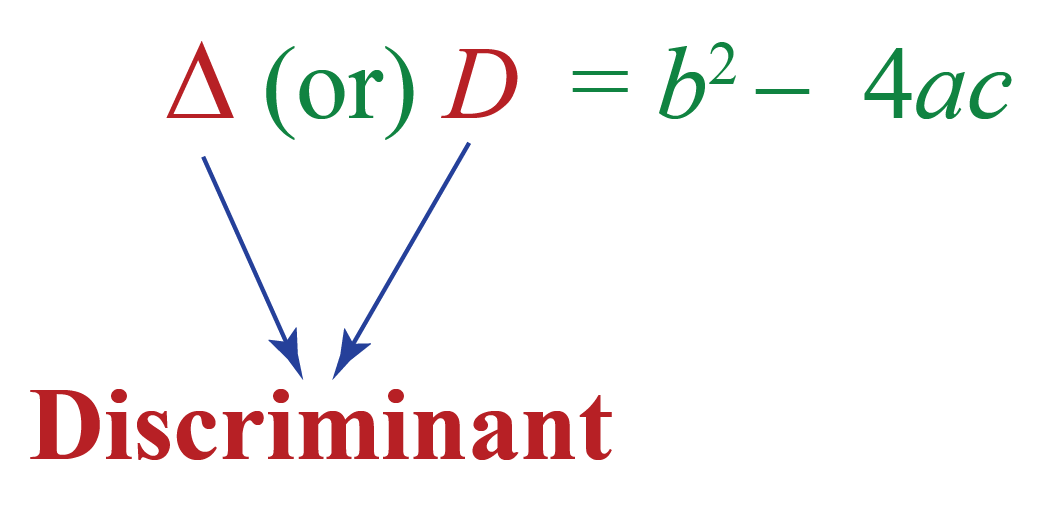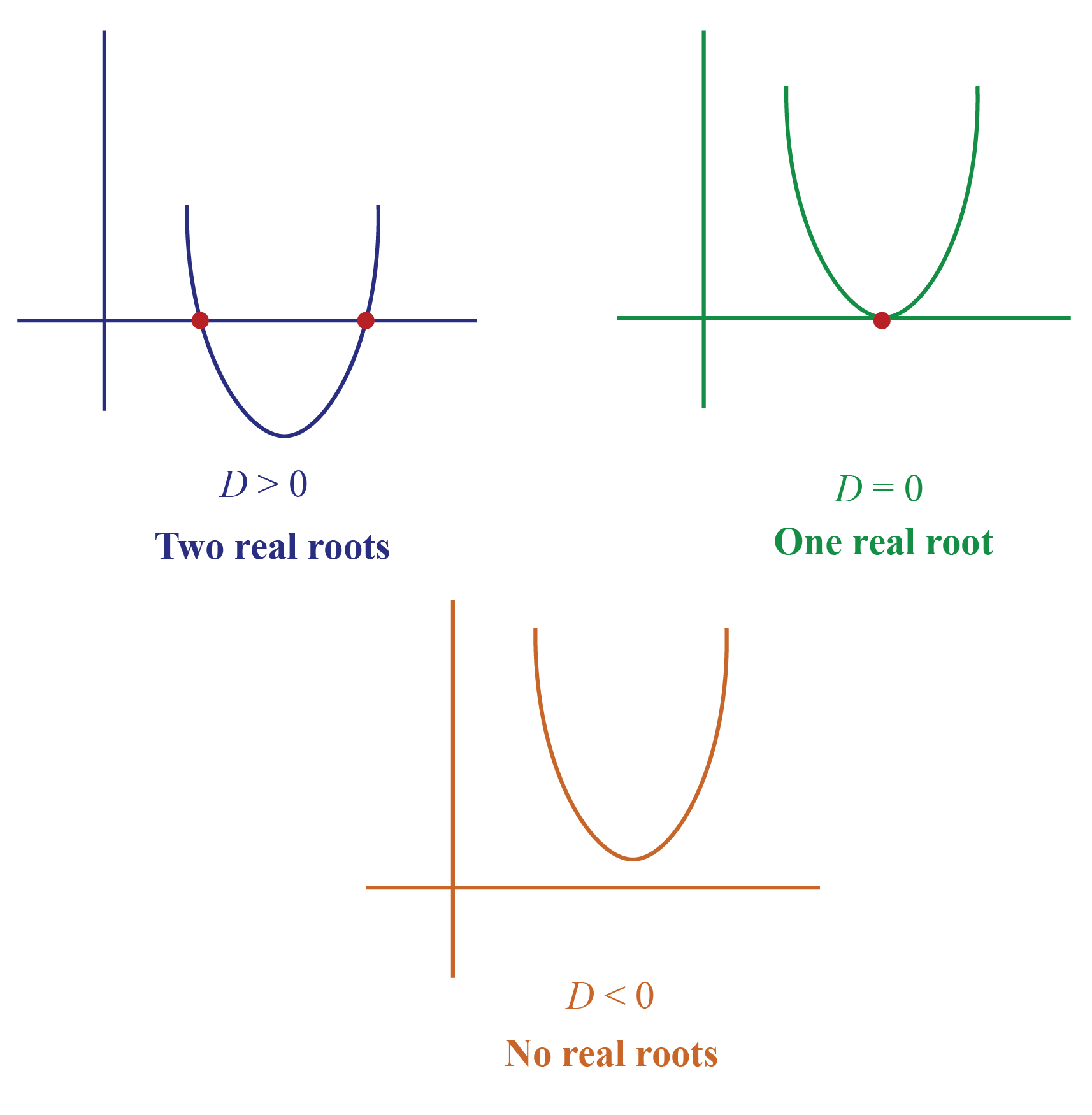# Discriminant

Discriminant

 1 Discriminant in Math 2 Discriminant Formula 3 Relation between Discriminant & Nature of Roots 4 Discriminant Calculator (with Graph) 5 Important Notes on Discriminant 6 Solved Examples on Discriminant 7 Practice Questions on Discriminant 8 Challenging Questions on Discriminant 9 Maths Olympiad Sample Papers 10 Frequently Asked Questions (FAQs)

We at Cuemath believe that Math is a life skill. Our Math Experts focus on the “Why” behind the “What.” Students can explore from a huge range of interactive worksheets, visuals, simulations, practice tests, and more to understand a concept in depth.

Book a FREE trial class today! and experience Cuemath’s LIVE Online Class with your child.

## Discriminant in Math

Discriminant in math is a function of the coefficients of the polynomial.

### Discriminant Symbol

The symbol of discriminant is either $$\mathbf{D}$$ or $$\mathbf{\Delta}$$

For example,

• the discriminant of a quadratic equation $$ax^2+bx+c=0$$ is in terms of $$a,b,$$ $$\text{ and }$$ $$c$$.
$\Delta \text{ OR } D=b^2-4ac$
• the discriminant of a cubic equation $$ax^3+bx^2+cx+d=0$$ is in terms of $$a,b,c$$ $$\text{ and }$$ $$d$$.
$\Delta\!\!=\!b^{2} c^{2}\!\!-\!4 a c^{3}\!\!-\!4 b^{3} d\!\!-\!27 a^{2} d^{2}\!\!+\!\!18 a b c d$## Discriminant Formula

The discriminant,  $$\mathbf{D}$$ or $$\mathbf{\Delta}$$, of a quadratic equation $$ax^2+bx+c=0$$ is:

 $$\mathbf{D}$$ or $$\mathbf{\Delta=b^{2}-4 a c}$$

Do you recall using $$b^2-4ac$$ earlier?

Yes, it is a part of the quadratic formula:

$x=\dfrac{-b \pm \sqrt{b^{2}-4 a c}}{2 a}$

Here, the expression that is inside the square root is called the discriminant of the quadratic equation.

The quadratic formula in terms of the discriminant is:

$x=\dfrac{-b \pm \sqrt{D}}{2 a}$

The discriminant can be either positive or negative or zero.

### Discriminant Examples

Here is a discriminant example.

Example

Find the discriminant of $$2x^2+3x+3=0$$

Solution

Compare the given expression with $$ax^2+bx+c=0$$,

$a=2\\b=3\\c=3$

The discriminant of the given equation is:

\begin{aligned} D \text{OR}\Delta &= b^2-4ac\\[0.2cm] &= 3^2 -4(2)(3)\\[0.2cm] &=9-24\\[0.2cm] &=-15 \end{aligned}

Thus, the discriminant of the given equation is:

 $$\mathbf{D}$$ or $$\mathbf{\Delta=-15}$$

## Relation between Discriminant and Nature of the Roots

The roots of a quadratic equation $$ax^2+bx+c=0$$ are the values of $$x$$ that satisfy the equation.

They can be found using the quadratic formula:

 $$x=\dfrac{-b \pm \sqrt{D}}{2 a}$$

Though we cannot find the roots by just using the discriminant, we can determine the nature of the roots as follows:

• If $$D>0$$, the quadratic equation has two different real roots:
$\dfrac{-b \pm \sqrt{ \text{Positive number}}}{2 a}$
gives two roots

• If $$D=0$$, the quadratic equation has only one real root:
$\dfrac{-b \pm \sqrt{0}}{2 a}= \dfrac{-b }{2 a}$
is the only root

• If $$D<0$$, the quadratic equation has no real roots. i.e., it has two complex roots:
$\dfrac{-b \pm \sqrt{ \text{Negative number}}}{2 a}$
gives two complex roots.

This is because the square root of a negative number leads to an imaginary number. i.e., $$\sqrt{-1} = i$$

A root is nothing but the x-coordinate of the x-intercept.

The graph of a quadratic equation in each of these 3 cases can be as follows.## Discriminant Calculator (with Graph)

Here is the "Discriminant Calculator".

You can move the sliders to change the coefficients of a quadratic expression.

Then it shows the corresponding discriminant.and the graph.

It also shows the number of roots depending on the discriminant.

More Important Topics
Numbers
Algebra
Geometry
Measurement
Money
Data
Trigonometry
Calculus
More Important Topics
Numbers
Algebra
Geometry
Measurement
Money
Data
Trigonometry
Calculus